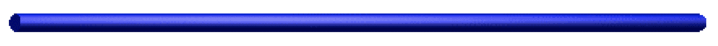﻿ Rejniak Moffitt Research Lab
Rejniak Lab

## Regularized Stokeslets Method for microPD:

A regularized Stokeslets framework for modeling drug pharmacodynamics at the micro-scale level.

The regularized Stokeslets method captures interactions between either stiff or elastic bodies (here: cells) and a surrounding incompressible viscous fluid (here: the interstitial fluid) at the low Reynolds numbers.

Regularized Stokeslets equations of the interstitial fluid flow: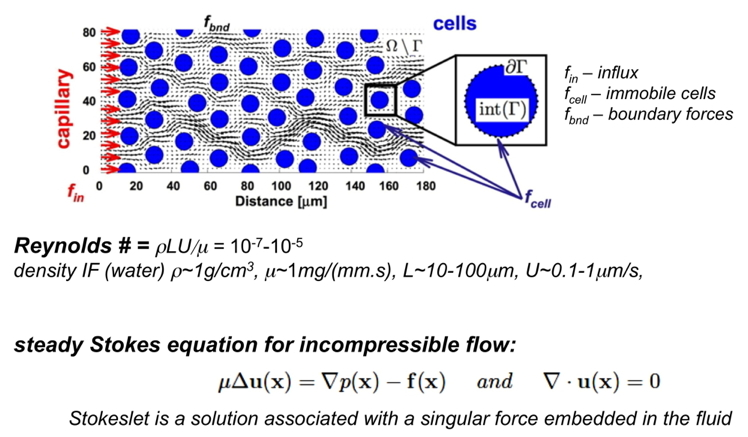In this model the fluid flow is approximated by the Stokes equations (above), where p is the pressure, u is the fluid velocity and f is the force applied to create physilogically relevant interstitial fluid flow-here f combines f_in applied to the left boundary (vessel) to create oxygen and drug influx; f_bnd applied to domain boundaries to provide no-slip conditions and f_cell to keep all cells immobile.

In the Regularized Stokeslets Method each force f concentrated at a single point x0 is smoothed over a small ball of radius δ using a cut-off (blob) function φ_δ that is f(x)=f0.φ_δ(x-x0). The cut-off function needs to be radially symmetric, vary smoothly from its maximal value at the center to zero at the surface, and satisfy the condition: ∫φ_δ(x-x0)dx=0. When the cut-off (blob) function is chosen properly (like the one shown below), the regularized Stokeslet equations have the exact solution, and since the Stokes equations are linear, one may represent the fluid flow as a direct summation of the contributions from finitely many discrete forces fk, shown below.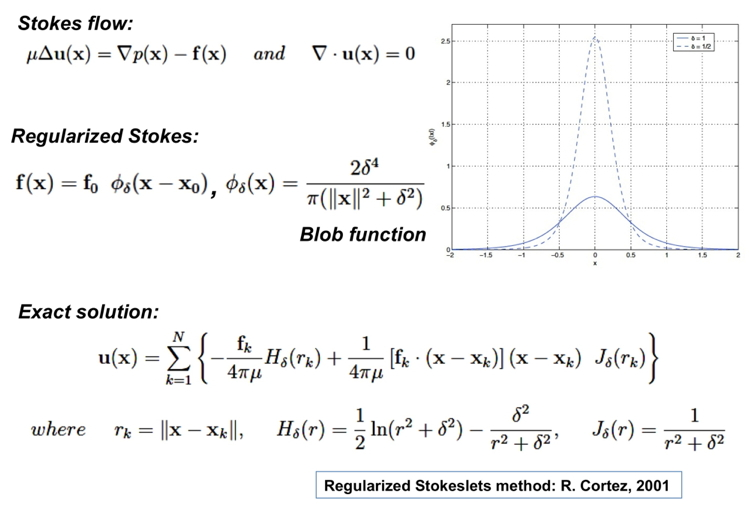Moreover, one can use iterative process, such as GMRES, to calculate forces that need to applied on the domain and cell boundaries in order to produce the desired boundary velocities. We calulated forces (red arrows below) that keep all cells immobile (velocity zero) when the fluid flow of a prescribed velocity is aupplied form the left domain boundary, adn the non-slip conditions are imposed on the top and bottom domain boundaries.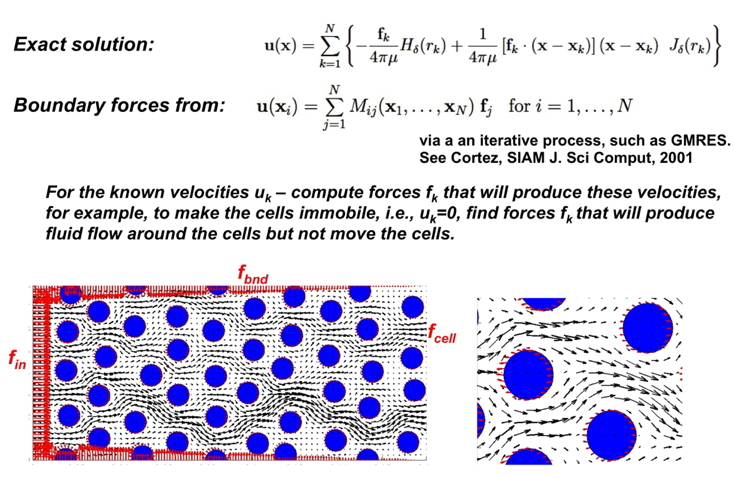The individual molecules of an imaging agents have been modeled as a collection of discrete particlesthat enter the tissue via the transmural influx from a capillary (left edge of the domain) together with the interstitial fluid, and advance through the tissue by a combination of advective transport, with the fluid flow calculated using the regularized Stokeslets method, and diffusion modeled as Brownian motion. The particle locations have been rendered to imitate their fluorescence.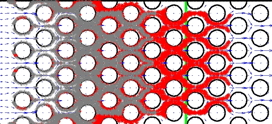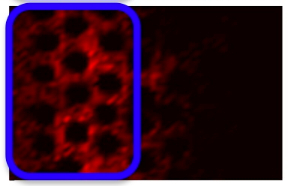More detail on the regularized Stokeslets method for the microPD model, its numerical implementation and solution can be found in:

• K.A. Rejniak, V. Estrella, T. Chen, A.S. Cohen, M.C. Lloyd, D.L. Morse, "The role of tumor tissue architecture in treatment penetration and efficacy: an integrative study", Frontiers in Oncology, 2013, 3:111 available on: journal website